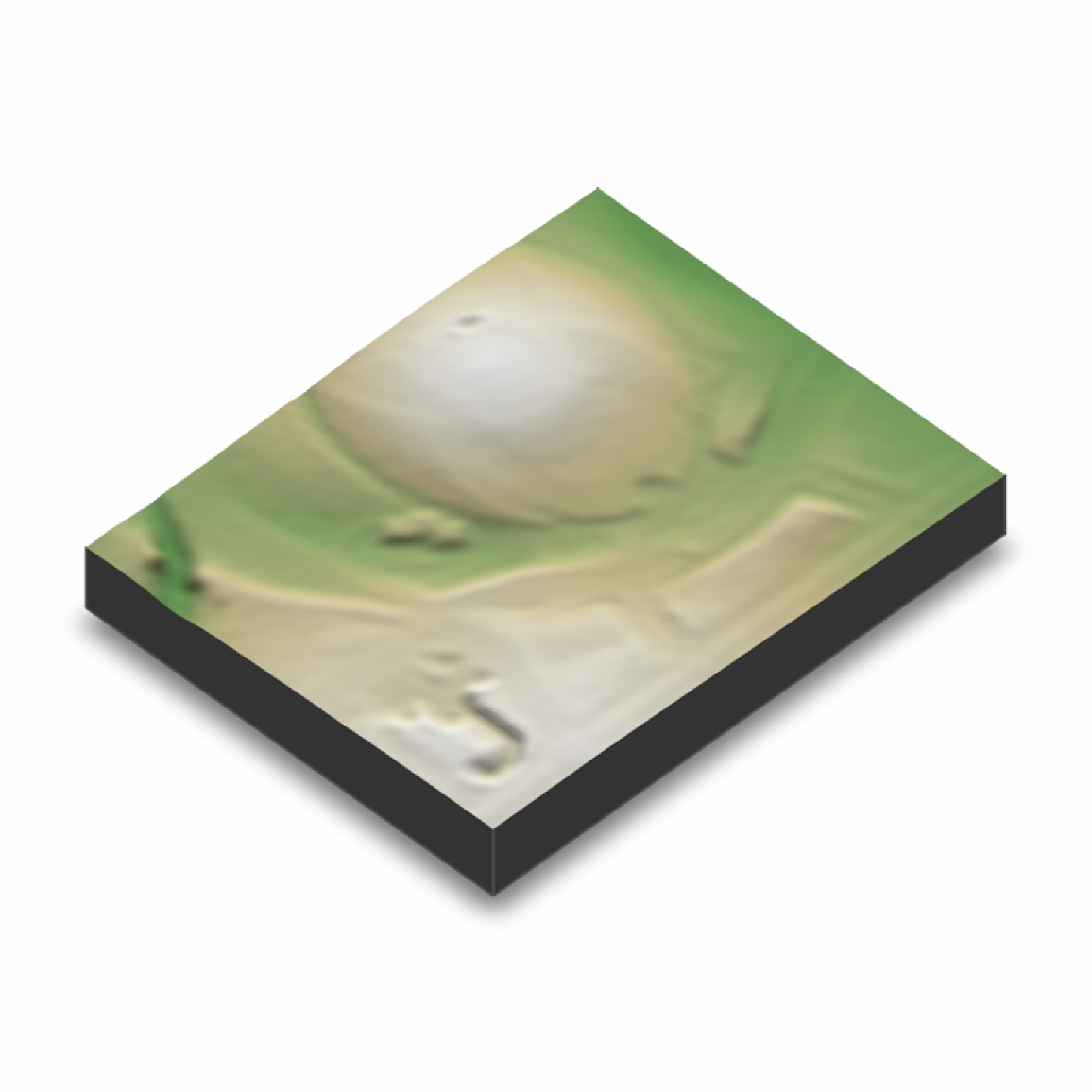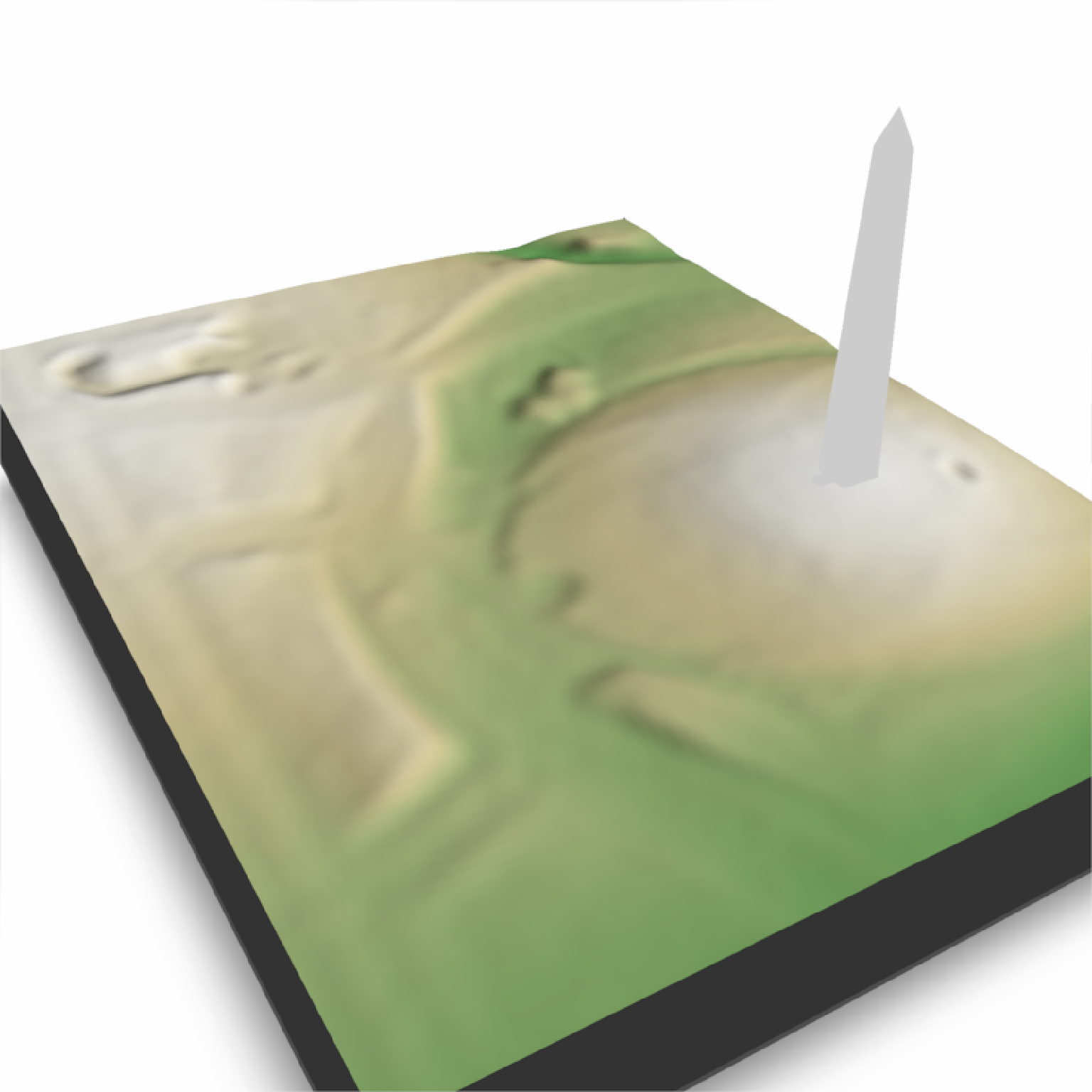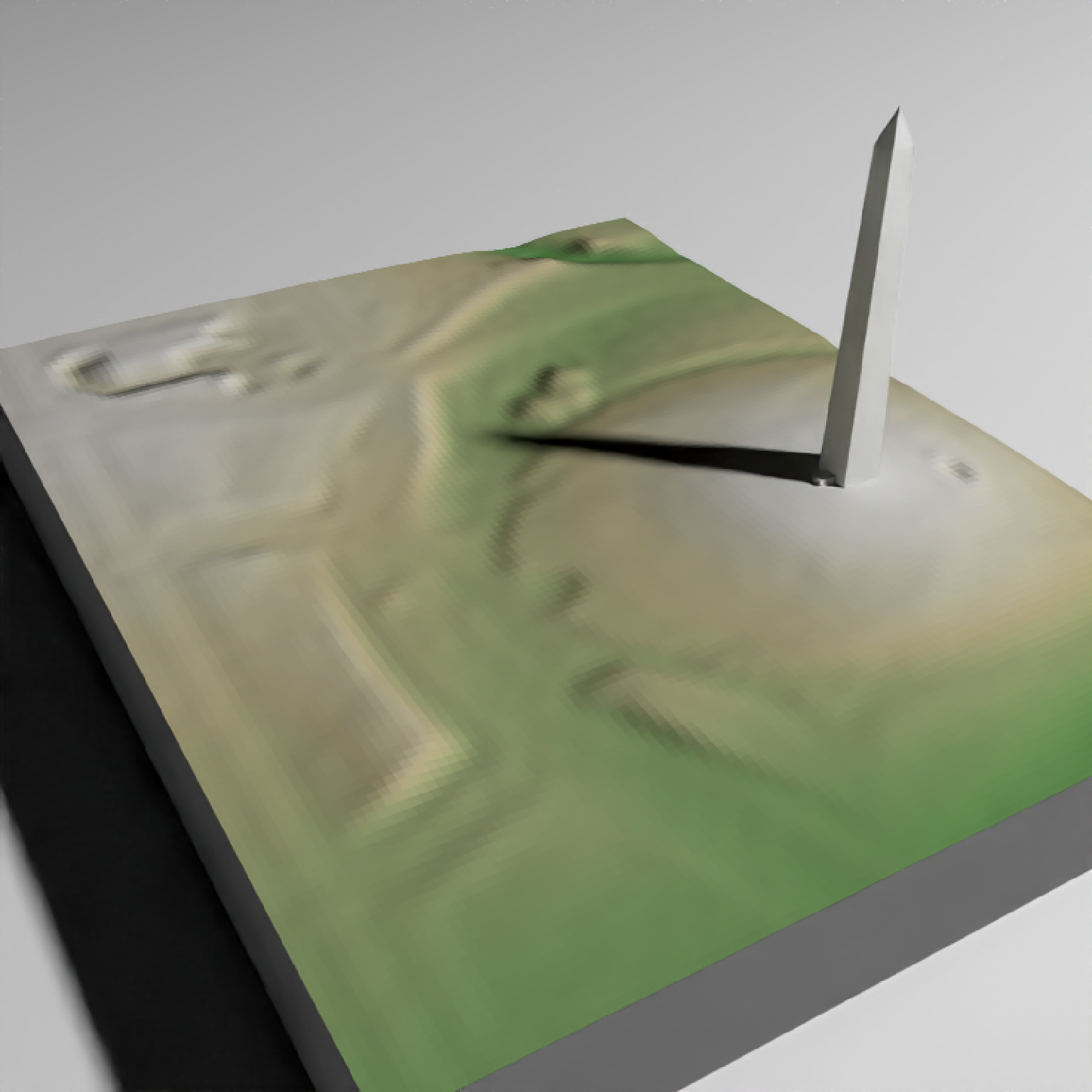Adds MULTIPOLYGONZ will be plotted in the coordinate system set by the user-specified extent argument as-is.

You can also use save_multipolygonz_to_obj() manually to convert sf objects

render_multipolygonz(
sfobj,
extent = NULL,
zscale = 1,
heightmap = NULL,
color = "grey50",
offset = 0,
obj_zscale = TRUE,
swap_yz = TRUE,
clear_previous = FALSE,
baseshape = "rectangle",
rgl_tag = "_multipolygon",
...
)

## Arguments

sfobj

An sf object with MULTIPOLYGON Z geometry.

extent

Either an object representing the spatial extent of the scene (either from the raster, terra, sf, or sp packages), a length-4 numeric vector specifying c("xmin", "xmax","ymin","ymax"), or the spatial object (from the previously aforementioned packages) which will be automatically converted to an extent object.

zscale

Default 1. The ratio between the x and y spacing (which are assumed to be equal) and the z axis in the original heightmap.

heightmap

Default NULL. Automatically extracted from the rgl window--only use if auto-extraction of matrix extent isn't working. A two-dimensional matrix, where each entry in the matrix is the elevation at that point. All points are assumed to be evenly spaced.

color

Default black. Color of the 3D model, if load_material = FALSE.

offset

Default 5. Offset of the track from the surface, if altitude = NULL.

obj_zscale

Default TRUE. Whether to scale the size of the OBJ by zscale to have it match the size of the map. If zscale is very big, this will make the model very small.

swap_yz

Default TRUE. Whether to swap and Y and Z axes. (Y axis is vertical in rayshader coordinates, but data is often provided with Z being vertical).

clear_previous

Default FALSE. If TRUE, it will clear all existing points.

baseshape

Default rectangle. Shape of the base. Options are c("rectangle","circle","hex").

rgl_tag

Default "". Tag to add to the rgl scene id, will be prefixed by "obj"

...

Additional arguments to pass to rgl::triangles3d().

## Examples

run_examples = length(find.package("sf", quiet = TRUE)) &&
length(find.package("elevatr", quiet = TRUE)) &&
length(find.package("raster", quiet = TRUE)) &&
if(run_examples) {
library(sf)
#Set location of washington monument
washington_monument_location =  st_point(c(-77.035249, 38.889462))
wm_point = washington_monument_location |>
st_point() |>
st_sfc(crs = 4326) |>
st_transform(st_crs(washington_monument_multipolygonz))

elevation_data = elevatr::get_elev_raster(locations = wm_point, z = 14)

scene_bbox = st_bbox(st_buffer(wm_point,300))
cropped_data = raster::crop(elevation_data, scene_bbox)

#Use rayshader to convert that raster data to a matrix
dc_elevation_matrix = raster_to_matrix(cropped_data)

#Remove negative elevation data
dc_elevation_matrix[dc_elevation_matrix < 0] = 0

#Plot a 3D map of the national mall
dc_elevation_matrix |>
plot_3d(dc_elevation_matrix, zscale=3.7, water = TRUE, waterdepth = 1,
soliddepth=-50, windowsize = 800)
render_snapshot()
}
#> Linking to GEOS 3.10.2, GDAL 3.4.2, PROJ 8.2.1; sf_use_s2() is FALSE
#> Warning: PROJ support is provided by the sf and terra packages among others
#> Warning: PROJ support is provided by the sf and terra packages among others
#> Mosaicing & Projecting
#> Note: Elevation units are in meters.if(run_examples) {
#Zoom in on the monument
render_camera(theta=150,  phi=35, zoom= 0.55, fov=70)
#Render the national monument
rgl::par3d(ignoreExtent = TRUE)
render_multipolygonz(washington_monument_multipolygonz,
extent = raster::extent(cropped_data),
zscale = 4, color = "white",
heightmap = dc_elevation_matrix)
render_snapshot()
}if(run_examples) {
#This works with render_highquality()
render_highquality(sample_method="sobol_blue", clamp_value=10, min_variance = 0)
}